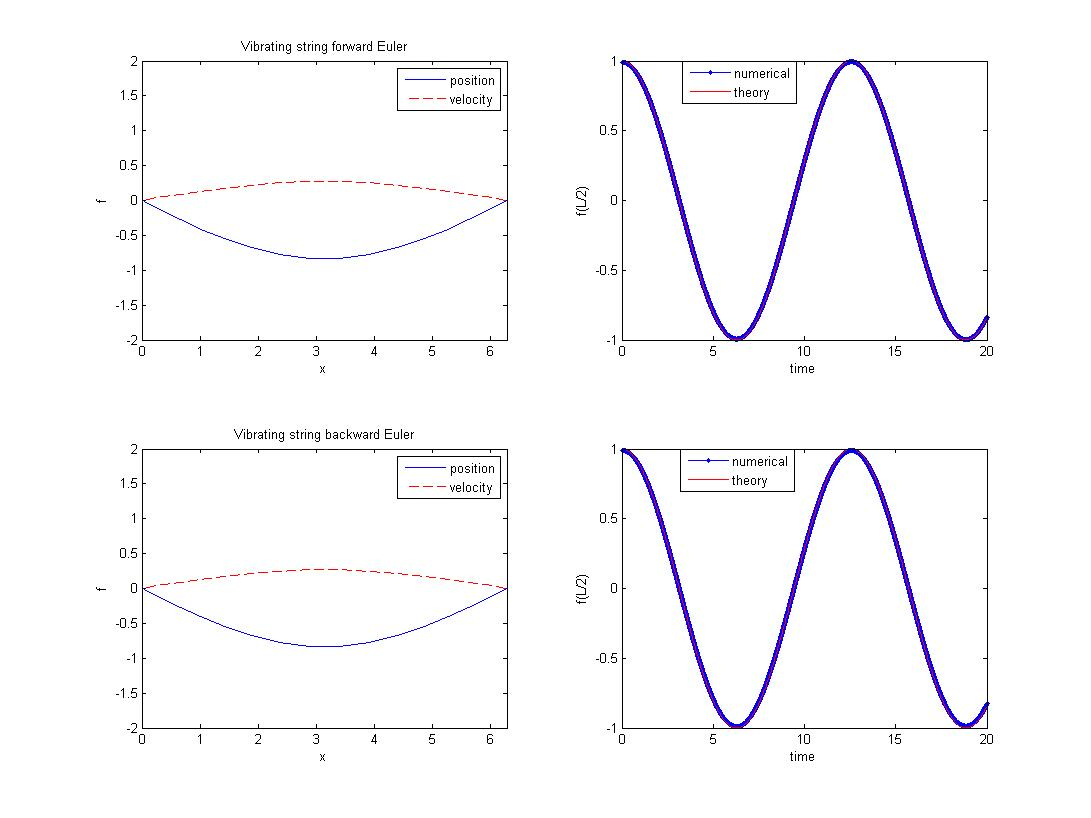# Forward Euler and Backward Euler scheme for the Vibration String

The reference source is /sandbox/easystab/vibrating_string.m , if you know it already, you can skip to March in time of the vibration string section.

We want to replace the Crank-Nicolson march in time scheme by Forward Euler and Backward Euler to compare. We thus, approximate the time integral of the right hand side by multiplying Aq(t) by the time step –> this will give us an explicit scheme. For the Backward Euler scheme, we will approximate the time integral with Aq(t+dt).

clear all; clf

% parameters
N=20; % number of gridpoints
L=2*pi; % domain length
c=1; % wave velocity
dt=0.002; % time step
tmax=20; % final time

% differentiation matrices
scale=-2/L;
[x,DM] = chebdif(N,2);
dx=DM(:,:,1)*scale;
dxx=DM(:,:,2)*scale^2;
x=(x-1)/scale;
Z=zeros(N,N); I=eye(N);
II=eye(2*N);

% system matrices
E=[I,Z; Z,I];
A=[Z,c^2*dxx; I, Z];

% locations in the state
v=1:N;
f=N+1:2*N;


# Boundary conditions

The original system has one variable and two time derivatives, so we should apply two boundary conditions. We will simply say that the position of the string at its both ends should be zero: two attachment points. This is a homogeneous Dirichlet condition applied on the first and last point of the state vector for the state position.

But there is one problem with the matrix E. As you will see in the next part, we construct the march-in-time matrix by using the inverse of matrix E. But if we impose the boundary condition as we do in the original code, matrix E will be non invertible (cause it has one row of 0).

To solve this problem, we will use the zero derivative boundary condition instead of the homogenous Dirichlet condition. This will give us the same result thanks to the characteristic of the Runge Kutta scheme. This scheme is a forward scheme, it means that it will calculate the value of the function at time t by the previous value at time t−dt. So if we impose the derivative in time of the function is zero and the initial condition is zero, we will always have the value of the function at that points is equal to zero independent of time t which means we have the homogenous Dirichlet condition.

As a result, we can impose the boundary condition like below:

% boundary conditions
loc=[f(1),f(N)];
A(loc,:)=0;
E(loc,:)=II(loc,:);


# March in time with the forward Euler scheme

To write a relation between the state at a given time and the state a little later, we will do a numerical approximation of the time derivative. We just integrate in time the evolution equation from time t to time t+dt \displaystyle \int_t^{t+dt} E q_t dt=\int_t^{t+dt} Aq dt the integral on the left hand side is just E(q(t+dt)-q(t)) since E does not change in time in this example, and the right hand side we approximate by multipling it by the time step \displaystyle E(q(t+dt)-q(t))\approx A q(t)dt since now we want to express the new state q(t+dt) as a function of the old state q(t), we rearrange \displaystyle Eq(t+dt)\approx (A dt + E) q(t) and we build the march-in-time matrix by inverting the left hand side matrix to transform this implicit system into an explicit system \displaystyle q(t+dt)\approx (A dt + E)q(t)/E This matrix inverse is well-defined since we have already imposed the propoer boundary conditions.

% march in time matrix for the forward scheme
M = E\(A*dt+E);


# March in time with the backward Euler scheme

Not like in the forward Euler scheme, we don’t encounter the problem about the non invertible matrix E because we won’t use the inverse of matrix E. As a result of that, we will impose the boundary condition as we do in the original code.

And now, we will see how to construct the march-in-time matrix for the backward Euler scheme

\displaystyle \int_t^{t+dt} E q_t dt=\int_t^{t+dt} Aq dt the integral on the left hand side is just E(q(t+dt)-q(t)) since E does not change in time in this example, and the right hand side we approximate by multipling it by the time step \displaystyle E(q(t+dt)-q(t))\approx A q(t+dt)dt \displaystyle q(t+dt)\approx (E-Adt)^{-1}Eq(t)

% boundary conditions
loc=[f(1),f(N)];
E(loc,:)=0;
A(loc,:)=II(loc,:);

% march in time matrix for the backward scheme
M2=(E-A*dt)\E;


# Initial condition

We need to describe the state of the system at initial time. We say here that the string is initially deformed as a sinus (this satisfies the boundary conditions), and that the velocity is zero. From that state we then perform a loop to repeatedly advance the state of a short forward step in time. We use drawnow to show the evolution of the simulation as a movie when running the code. We store for validation the string position at the midle of the domain, to do this without worrying about the the grid points are, we interpolate f with the function interp1.

% initial condition
q=[zeros(N,1); sin(pi*x/L)];

% marching loop
tvec=dt:dt:tmax;
Nt=length(tvec);
e=zeros(Nt,1);

for ind=1:Nt
q=M*q; % one step forward
ef(ind)=interp1(x,q(f),L/2); % store center point
end

% plotting
subplot(2,2,1);
plot(x,q(f),'b',x,q(v),'r--');
axis([0,L,-2,2])
drawnow

legend('position','velocity'); title('Vibrating string forward Euler')
xlabel('x'); ylabel('f');

subplot(2,2,2);
Ttheo=2*L/c;
tt=linspace(0,tmax,500);
etheo=cos(2*pi*tt/Ttheo);
plot(tvec,ef,'b.-',tt,etheo,'r-');
legend('numerical','theory');
xlabel('time'); ylabel('f(L/2)');

% initial condition
q=[zeros(N,1); sin(pi*x/L)];

% marching loop
tvec=dt:dt:tmax;
Nt=length(tvec);
e=zeros(Nt,1);

for ind=1:Nt
q=M2*q; % one step forward
eb(ind)=interp1(x,q(f),L/2); % store center point
end

% plotting
subplot(2,2,3);
plot(x,q(f),'b',x,q(v),'r--');
axis([0,L,-2,2])
drawnow

title('Vibrating string backward Euler')
% time evolution of central point

subplot(2,2,4);
Ttheo=2*L/c;
tt=linspace(0,tmax,500);
etheo=cos(2*pi*tt/Ttheo);
plot(tvec,eb,'b.-',tt,etheo,'r-');
legend('numerical','theory');
xlabel('time'); ylabel('f(L/2)');


# ValidationValidation

To check the error for those schemes are of order 1 you can check the page of alexandre bilczewski right here –>vibrating_string_convergence_time_euler_backward.m